StatLect

# Factorial

The factorial of a natural numberis the product of all natural numbers smaller than or equal to.

On this page we provide a basic introduction to factorials and we explain how they are used in probability theory and statistics.## Definition

The following is a formal definition.

Definition Let. The factorial of, denoted by, is: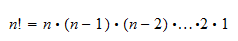The expressionis read "factorial".

This definition is extended to the number 0 by using the convention:## Examples

For example, the factorial of 6 is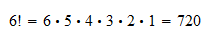It is frequent to encounter ratios of factorials, which can be computed by simplifying the common terms. For example,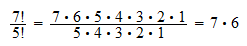## Use in probability

In the calculus of probabilities we often need to count permutations, combinations and partitions of objects. This can easily be done with factorials.

### Counting permutations

A permutation is one of the possible ways of orderingobjects, from first to last.

The number of possible permutations is equal to.

Example Consider the first three letters of the alphabet:. There are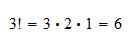ways of ordering these letters:### Counting combinations

A combination is a way of selectingobjects from a list of. The order of selection does not matter and each object can be selected only once.

The number of possible combinations is equal toExample The number of possible ways to choose a team of three people from a group of five is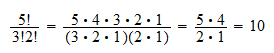### Counting partitions

A partition is a way of subdividingobjects intogroups having numerosities.

The number of possible partitions is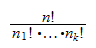Example The number of possible ways to assign six individuals to three teams of two people is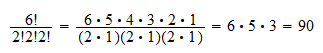## Use in statistics

Factorials have numerous important applications in the analysis of probability distributions.

For example, they appear in the probability mass functions of:

## Generalization

The concept of factorial can be extended using the Gamma function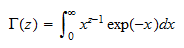Unlike the factorial, the Gamma function is defined also whenis not an integer.

It has the property that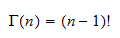whenis an integer.

The Gamma function is often used in statistics, for example, in the probability density functions of:

In turn, the Gamma function is used to define the Beta function, which is found in the densities of

## More details

An in-depth explanation of factorials can be found in the lecture entitled Permutations.

Previous entry: Event

Next entry: Heteroskedasticity